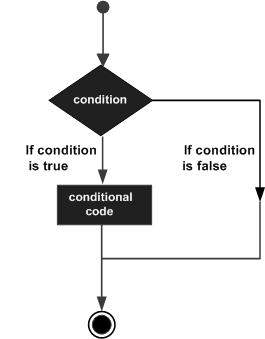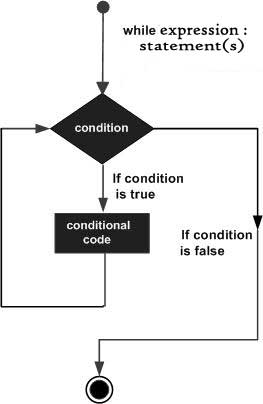# Python教程-5. 控制语句

## control_flow

Posted by xuepro on November 15, 2017

## 控制语句（control_flow）

### 1. if条件语句

• 1） 按照python语法，条件语句块要缩进

• 2） 条件表达式后要跟一个冒号’:’ 。

if 条件真：
条件语句块
...if 条件真：      #如果条件真，执行“条件语句块”
条件语句块
else             #否则，执行“条件语句块2“
条件语句块2
...


if 条件1：         #如果条件1为真，执行“条件1语句块”
条件1语句块

elif 条件2         #否则如果条件2为真，执行“条件2语句块”
条件2语句块

elif 条件3         #否则如果条件3为真，执行“条件3语句块”
条件3语句块
else              #否则(其他情况)，执行“其他情况语句块”
其他情况语句块
...


例如下面的程序：

>>> x = int(input("Please enter an integer: "))
>>> if x < 0:
...     x = 0
...     print('Negative changed to zero')
... elif x == 0:
...     print('Zero')
... elif x == 1:
...     print('Single')
... else:
...     print('More')
...
More


var = 100
if var < 200:
print "Expression value is less than 200"
if var == 150:
print "Which is 150"
elif var == 100:
print "Which is 100"
elif var == 50:
print "Which is 50"
elif var < 50:
print "Expression value is less than 50"
else:
print "Could not find true expression"

print "Good bye!"


Expression value is less than 200
Which is 100
Good bye!


### 2. while条件语句

while 条件真：
语句块
...count = 0
while (count < ):
print 'The count is:', count
count = count + 1


The count is: 0
The count is: 1
The count is: 2
The count is: 3


### 3. for条件语句

for 迭代变量 in 可迭代序列：
语句块


#输出字符串'Python'中的每一个元素，即字符

for letter in 'Python':    # 字符串'Python'是一个可迭代序列，letter是其中的每一个迭代变量，即其每一个字符
print 'Current Letter :', letter

#输出列表中的每一个元素

fruits = ['banana', 'apple',  'mango']   # 列表是一个可迭代变量
for fruit in fruits:        # Second Example
print 'Current fruit :', fruit


P
y
t
h
o
n
banana
apple
mango


range()可以用来生成一个可迭代的整数序列。例如

class range(stop)
class range(start, stop[, step])


>>> list(range(10))
[0, 1, 2, 3, 4, 5, 6, 7, 8, 9]
>>> list(range(1, 11))
[1, 2, 3, 4, 5, 6, 7, 8, 9, 10]
>>> list(range(0, 30, 5))
[0, 5, 10, 15, 20, 25]
>>> list(range(0, 10, 3))
[0, 3, 6, 9]
>>> list(range(0, -10, -1))
[0, -1, -2, -3, -4, -5, -6, -7, -8, -9]
>>> list(range(0))
[]
>>> list(range(1, 0))
[]


>>> for i in range(5):
...     print(i)
...
0
1
2
3
4


>>> a = ['Mary', 'had', 'a', 'little', 'lamb']
>>> for i in range(len(a)):
...     print(i, a[i])
...
0 Mary
2 a
3 little
4 lamb


>>>#打印range生成的对象
>>> print(range(10))
range(0, 10)

>>># list 将range生成的对象转化为了一个列表
>>> list(range(5))
[0, 1, 2, 3, 4]


### 4. 循环体中的break、continue、else

>>> for n in range(2, 10):
...     for x in range(2, n):
...         if n % x == 0:
...             print(n, 'equals', x, '*', n//x)
...             break
...     else:
...         # loop fell through without finding a factor
...         print(n, 'is a prime number')
...
2 is a prime number
3 is a prime number
4 equals 2 * 2
5 is a prime number
6 equals 2 * 3
7 is a prime number
8 equals 2 * 4
9 equals 3 * 3


continue和C语言一样，用于循环体中，一旦遇到continue语句，则循环体其余语句不再执行，而回到循环体开头，继续循环。 例如：

>>> for num in range(2, 10):
...     if num % 2 == 0:
...         print("Found an even number", num)
...         continue
...     print("Found a number", num)
Found an even number 2
Found a number 3
Found an even number 4
Found a number 5
Found an even number 6
Found a number 7
Found an even number 8
Found a number 9


### 5. pass条件语句

pass语句什么也不做，它用在那些语法上至少组要一个语句但实际不需要做任何事情的地方。 有时它用作“占位符”：就是写程序时，还没有想好代码，暂时先空着，等以后再补充代码的地方

if __name__ == "__main__":
pass


>>> def initlog(*args):
...     pass   # 暂时空着，记得以后这里要补充代码哦
...


class MyClass(object):
def meth_a(self):
pass

def meth_b(self):
print "I'm meth_b"